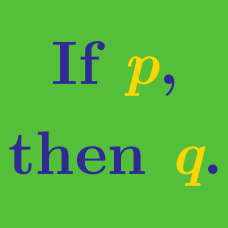Logic

# Operator Search Warmup

What sign, in place of $\square$, will make the equation true?

$\large 6 \square 2 = 4$

Using only $+$ and $-$, can we fill in the boxes to make the following expression true:

$\large 6 \square 4 \square 2 = 8 ?$

Out of the 4 standard arithmetic operations ($+, - , \times, \div$), can we place them into $\square$ such that the equation is true?

$\large 1 \square 2 \square 3 = 6$

Note: Each $\square$ could use the same or a different operation.

Using only $+$ and $-$, can we fill in the boxes to make the following expression true:

$\large 1 \square 2 \square 3 \square 4 \square 5 = 10 ?$

Out of the 4 signs ($+, -, \times, \div$), how many of them could be placed in $\square$ to make the statement true?

$\large 2 \square 2 = 4$

×